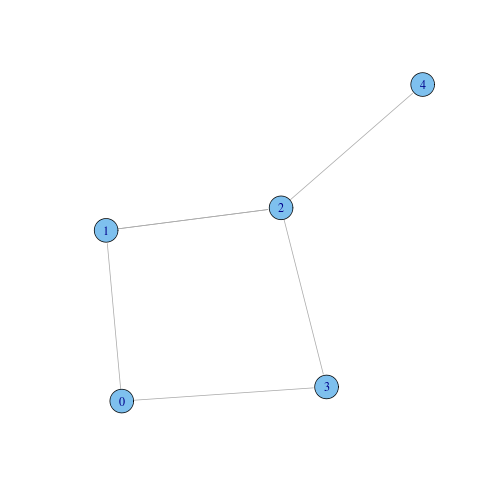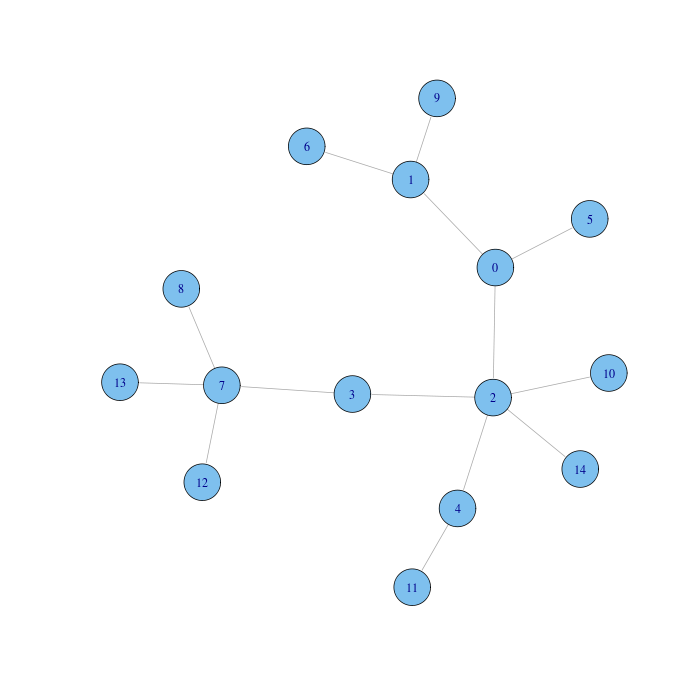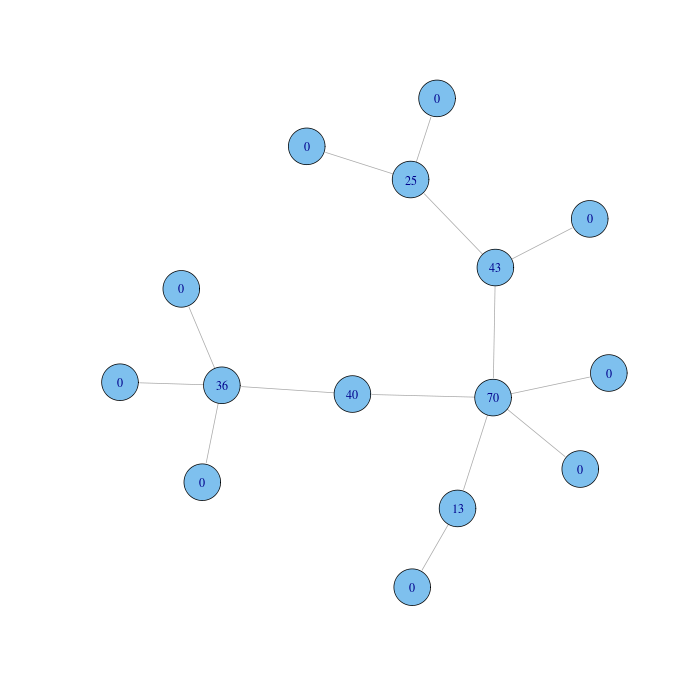# Betweenness Centrality

Betweenness centrality measures the extent to which a vertex lies on paths between other vertices. Vertices with high betweenness may have considerable influence within a network by virtue of their control over information passing between others. They are also the ones whose removal from the network will most disrupt communications between other vertices because they lie on the largest number of paths taken by messages.

Mathematically, let $n_{s,t}^{i}$ be the number of geodesic paths from $s$ to $t$ that pass through $i$ and let $n_{s,t}$ be the total number of geodesic paths from $s$ to $t$. Recall that a geodesic path is not necessarily unique and the geodesic paths between a pair of vertices need not be node-independent, meaning they may pass through some of the same vertices. Then the betweenness centrality of vertex $i$ is:

$\displaystyle{b_i = \sum_{s, t} w_{s,t}^{i} = \sum_{s, t} \frac{n_{s,t}^{i}}{n_{s,t}}}$
where by convention the ratio $w_{s,t}^{i} = 0$ if $n_{s,t} = 0$. Notice that each pair of vertex $s, t$ contribute to the sum for $i$ with a weight $w_{s,t}^{i}$ between 0 and 1 expressing the betweenness of $i$ with respect to the pair $s, t$. Observe that:

1. the given definition counts separately the geodesic paths in either direction between each vertex pair. Since these paths are the same on an undirected graph this effectively counts each path twice;
2. the definition includes paths starting or ending with $i$ ($s$ can be equal to $i$ or $t$ can be equal to $i$), as well as paths from a vertex to itself ($s$ can be equal to $t$). It seems reasonable to define a vertex to be on a path between itself and someone else, or between some vertex and itself, since normally a vertex has control over information flowing from or to itself.

It makes little difference in practice to consider the alternative definitions, since one is usually concerned only with the relative magnitudes of the centralities and not with their absolute values. The sum can be normalized by dividing by the total number of ordered pairs of nodes, which is $n^2$, so that betweenness lies strictly between 0 and 1.

Consider the following graph:From node 0 to node 4 there are two geodesics, both going through node 2. Hence $w_{0,4}^{2} = 2 / 2 = 1$, $w_{0,4}^{1} = w_{0,4}^{3} = 1 / 2$.

Consider once again the following simple network:Function betweenness (R, C) computes betweenness centrality (the function counts undirected paths in only one direction and computes the sum in the betweenness formula for $s \neq t$, $s \neq i$ and $t \neq i$). This is the same network with nodes labelled with their betweenness centrality:Notice that our sample graph is in fact a tree, that is a connected acyclic undirected graph, hence the number $n_{s,t}$ of geodesics from $s$ to $t$ is always 1, and the number $n_{s,t}^{i}$ of geodesics from $s$ to $t$ that pass through $i$ is either 0 or 1. Hence the computed betweenness score for a vertex is the actual number of distinct paths that strictly contain the vertex in between.

Betweenness centrality differs from the other centrality measures. A vertex can have quite low degree, be connected to others that have low degree, even be a long way from others on average, and still have high betweenness. Consider a vertex A that lies on a bridge between two groups of vertices within a network. Since any path between nodes in different groups must go through this bridge, node A acquires high betweenness even though it is not well connected (it lies at the periphery of both groups) and hence it might not have particularly high values for degree, eigenvector, and closeness centrality. Vertices in roles like this are sometimes referred to as brokers.

The maximum possible value for betweenness occurs for the central node of a star graph, a network composed of a vertex attached to $n-1$ other vertices, whose only connection is with the central node. All paths, except the $n-1$ paths from the peripheral vertices to themselves, go through the central vertex, hence its betweenness is $n^2 - (n-1) = n^2 - n + 1$. At the other end of the scale, the smallest possible value for betweenness occurs for a leaf node in a graph with a single component, that is a node that is connected to the rest of the network with only one edge. The leaf vertex lies on every path that starts or ends with itself. These are $2n-1$ in total: $n-1$ paths from a vertex to others, $n-1$ from others to the vertex, and 1 from the vertex to itself.

Betweenness centrality values are typically distributed over a wide range. Taking again the example of the film actor network, the individual with highest betweenness in the largest component of the network is Fernando Rey (The French Connection). It is no coincidence that he appeared in both European and American films, played roles in several languages, and worked in both film and television, hence he is the archetypal broker. Rey has a betweenness score of $7.47 \cdot 10^8$, while the lowest score of any actor in the large component is $8.91 \cdot 10^5$. Thus there is a ratio of almost a thousand between the two limits, much larger than the ratio of 3.6 we saw in the case of closeness. One consequence is that there are clear winners and losers in the betweenness centrality competition. The second highest betweenness score is attributed to Christopher Lee again, with $6.46 \cdot 10^8$, a $14%$ difference from the winner.

Betweenness centrality, as defined above, is a measure of information control assuming two important hypothesis: (i) every pair of vertices exchange information with equal probability, and (ii) information flows along the geodesic (shortest) path between two vertices, or one of such path, chosen at random, if there are several. However, information not always takes the shortest route. In social network, for instance, a news about a friend of us might not come directly from the friend but from another mutual friend.

Calculating closeness and betweenness centrality for all nodes in a graph involves computing the (unweighted) shortest paths between all pairs of nodes in the graph. A breath-first visit from a source node can be used to compute all shortest paths from the source in time $O(n + m)$, where $n$ and $m$ are the number of nodes and edges of the graph. If this visit is repeated for all the source nodes of the graph the cost amounts to $O(n \cdot (n+m))$. Typically, real networks are sparse graphs, meaning the number $m$ of edges is of the order of the number $n$ of nodes. For instance, it is very rare in a social network that a significant number of actors have contacts with all the other actors of the network. Hence the overall cost in practical cases is quadratic.